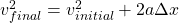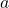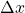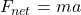# NASCAR Pile Up and Importance of Friction

A real life examples to help explain the importance of tribology are priceless. Car races can provide an exciting example. A pile up at the 2019 Daytona NASCAR car race created an example the effect of friction in our life.

On lap 190, with only nine laps to go, the car of Paul Menard tapped Matt DiBenedetto’s car, causing him to lose control and spin across the track. The result was a massive crash involving a total of 21 cars. The results are spectacular to watch, especially considering that all drivers safely got out of their cars. Given the spectacle of the crash and the lack of serious injuries, it provides a perfect opportunity for a tribology lesson, a lesson relating frictional forces, velocity, and acceleration.

As the cars slide sideways across the racetrack, they experience frictional force, which occurs whenever two different objects slide against one another. In this case, it is the contact between the cars’ tires and the racetrack surface creating the frictional force. As the tires slide sideways, the friction converts kinetic energy (energy of motion) into thermal energy (energy of heat). This frictional force causes the car to slow down (take a look at this ultimate guide to car tires from CarBibles).The strength (or magnitude) of the frictional force depends on the normal force (how hard the car is pushed down against the racetrack) and the types of materials pushing against each other. This strength is called the coefficient of friction. To find this it is necessary first to determine the acceleration of the car.

One way to find the acceleration is to use the formula:whereandare the final and initial velocities,is the acceleration andis the distance traveled to the stop. The final velocity is zero and the initial velocity and time it took the car to come to a complete stop can be estimated from the video and Google. Rhett Allain made the necessary estimations for us (see the reference), so that we just take his values for a distance – 468 meters and initial speed – 84 meters per second. This gives an acceleration of -7.53 m/s2 .

Using the calculated acceleration, it is possible to find the coefficient of friction (or the strength of the friction) using following equations:Thus,This gives a coefficient of kinetic friction at 0.769. While there are other forces at work in the process, this approach provides a good first approximation for the friction coefficient. Pretty close to the values given in the source.

The kinetic friction coefficient of 0.769 corresponds to a dry asphalt – rubber tire situation. What would happen, if it would be a rainy day? The friction coefficient would drop to 0.4 and this would increase the distance traveled prior to stop. The acceleration would be -3.92 m/s2 and the distance traveled to stop would be 900 meters.

Now, the lesson here: from the equation linking the distance and the acceleration, no matter how large is friction coefficient, the increase of the speed 2 times, leads to an increase of the stopping distance 4 times! Driving in rainy conditions increases the stopping distance as well, since the friction drops. Bare that in mind!

Drive safely and keep faith in tribology.

The article is based on a story by Rhett Allain: “Estimate the Friction Coefficient in That Massive NASCAR Pile-Up” (www.wired.com/story/estimate-the-friction-force-in-that-massive-nascar-pile-up).

•
• 1
•
•